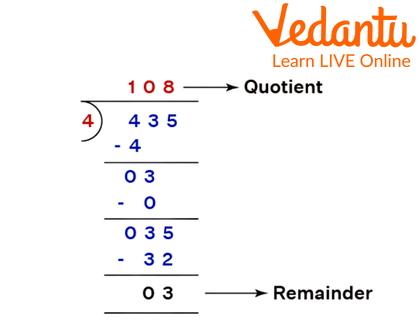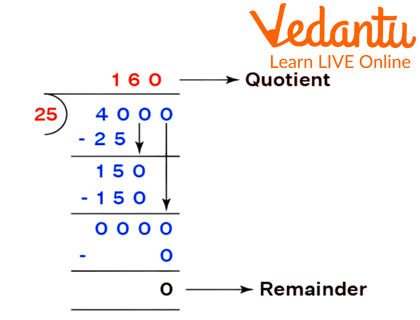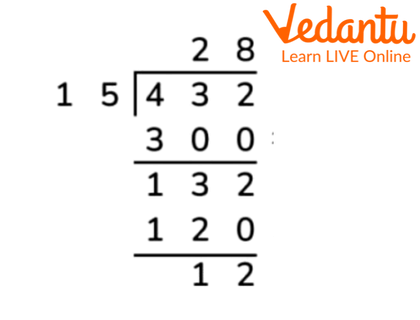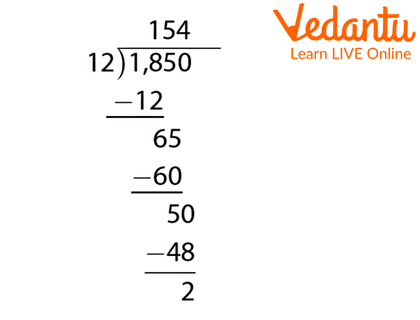Courses
Courses for Kids
Free study material
Offline Centres
More

# Long Division for Class 3Last updated date: 27th Nov 2023
Total views: 108k
Views today: 1.08k## Introduction to Long Division

Long division is a method in Mathematics for dividing big numbers into steps or parts, breaking the division questions into smaller stages. It is the most commonly used approach for dividing problems and makes it easier for kids to learn about the division better. Examine the following long division to find the divisor, dividend, quotient, and remainder. Before executing it, we must be aware of the crucial aspects of long division.

## Long Division with Single-Digit Divisor

Long division is done like simple division. When the divisor is a single digit, it is easy to divide the dividend. You can learn and practice the steps that need to be followed while long division is given.

Step One: Check the first unit of the dividend whether it is divisible by the divisor or not.

Step Two: If the first unit is less than the divisor, take the second digit into account.

Step Three: Then, find the suitable multiple and subtract the numbers. After subtraction brings down the next digit and then again repeat the above steps till you get the remainder as 0 or a number that further cannot be divided.Example of Long Division With Single Digit Divisor

## Long Division with Two-Digit Divisor

The long division with a two-digit divisor can be done using the same steps that are mentioned above. In this, the only change is that in the dividend we take the first two digits of the number to subtract it from the multiple of the divisor. And the rest of the steps are the same.Example of Long Division of Two- Digit Divisor

## Long Division with Remainders

The long division ends when there is a remainder left that cannot be further divided by the divisor or the value of the number is less than the divisor itself. Like in the above-mentioned 435 divided by 4 sample questions, after taking down the last digit 5 to be subtracted we are left with 3. As 3 is less than the divisor 4 so we will now conclude the long division as there are no more digits present in the dividend, which can be used to reduce it. So, we are left with 3 as the remainder.

## Long Division without Remainders

Long division without remainders means that the remainder is 0 or the last number to be subtracted by the multiple of the divisor was the same giving us the remainder 0. In an example of a division of 126 by 2, we will get 63 as the quotient. As the last digit 6 is completely divisible by 2 we get the remainder as 0 making it a long division without remainder.

## Conclusion

The long division is similar to the standard division. The division consists of dividend, divisor, quotient, and the remainder where the divisor can be single-digit, double-digit, or triple-digit. The remainder can be zero or a number that is less than the divisor. If the dividend does not get divided fully or does not have a remainder as 0, then the division can continue further by using a decimal point. The divisor can be single-digit, two-digit, or three-digit.

## Long Division Worksheets

1. Divide 432 by 15.

1. 27

2. 28

3. 29

4. 30

Ans: 28

Explanation: In this, we will take the first two digits of the dividend as our divisor is two digits. A multiple of 15 which is less than or equal to 43 is found and then it is subtracted. The remainder along with the next digit of the dividend is then evaluated and subtracted again.Solution of the division of 432 by 15

2. Divide 1850 by 12 using long division.

1. 144

2. 154

3. 164

4. 174

Ans: 154

Explanation: Step one: As 18 is a little greater than the divisor itself so we will subtract it by 12. We get 6 as the remainder.

Step Two: Bring down 5 and the new number becomes 65 which can be subtracted from 60 which is a multiple of 12.

Step Three: Now bring down 0 and subtract it with the multiple of 12. There is a remainder left as 2. We get our answer as 154 the quotient.Solution of Division of 1850 by 12

3. What is the value of the remainder when the division ends without any remainder left?

1. 1

2. 0

3. not defined

4. none of the above

Ans: 0

## FAQs on Long Division for Class 3

1. What is the polynomial long division?

Ans: Long division of polynomials is a method in algebra that divides a polynomial by another polynomial of the same or lower degree.

2. How do you do long division with decimals?

Ans: Long division with decimals is done in the same way as regular division. It is done in the following order:

• Fill in the blanks with the normal form of the division.

• To begin, divide the whole number component by the divisor.

• Place the quotient's decimal point above the dividend's decimal point.

• Bring down the numerals to the tenth place, that is, the digit after the decimal.

• Divide and bring down the next digit in order.

• Divide until all of the dividend digits are used up and the remainder has a value smaller than the divisor or 0.

3. What is a division used for?

Ans: Long division is used to find an answer to the numbers which need to be shared. In a case where you want to distribute 80 chocolates equally among your 8 friends, so you would use division in such a case.

4. State if the statement true or untrue. When a long division is used, the remainder is always less than the divisor.

Ans: True, in the case of long division, the remainder is always less than the divisor.

5. How is a long division better than a short division?

Ans: The shorter division method is used to divide large complex numbers by a single digit number, whereas the long division method is used to divide complex numbers by two or three-digit numbers.